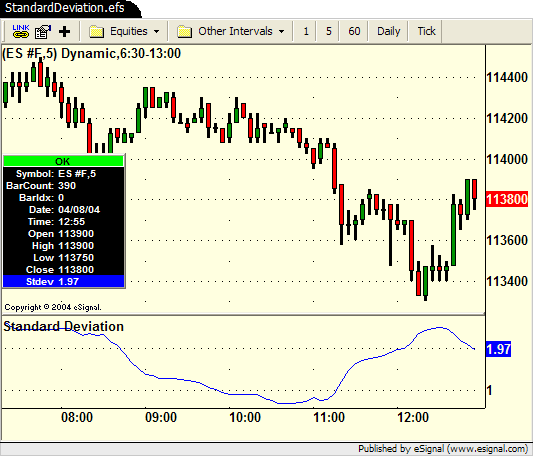# StandardDeviation.efs

ICE Data Services -

StandardDeviation.efs

File Name: StandardDeviation.efs

Description:

This formula is intended to be used within custom formulas through the callFunction() function to get the standard deviation of one of the following price sources (Open, High, Low, Close, Volume). It may also be used as a non-price study.

Formula Parameters:

• nLength - 25
• sPriceSource - "Close"

Notes:
Here's an example for calling this formula from a custom formula. If the formula is in a different folder than the calling formula, change the first input parameter to the correct path (i.e. "/Other/StandardDeviation.efs").

`var nStdev = callFunction("StandardDeviation", "main", 10, "Close");if (nStdev == null) return; // null check`

StandardDeviation.efsEFS Code:

```/*****************************************************************
Provided By : eSignal. (c) Copyright 2004
Study:  Standard Deviation

Version 1.0 - 4/8/204

Formula Parameters:     Defaults:
nLength             25
sPriceSource        "Close"

Notes:
This formula is intended to be used within custom formulas
through the callFunction() function to get the standard
deviation of one of the following price sources.  It may also
be used as a non-price study.
"Open"
"High"
"Low"
"Close"
"Volume"

Example Usage:
var nStdev = callFunction("StandardDeviation.efs", "main", 10, "Close");
if (nStdev == null) return;

*****************************************************************/

function preMain() {
setStudyTitle("Standard Deviation ");
setDefaultBarFgColor(Color.blue);
setCursorLabelName("Stdev");
}

function main(nLength, sPriceSource) {
if (nLength == null) nLength = 25;
if (sPriceSource == null) sPriceSource = "Close";

var aSource = getValue(sPriceSource, 0, -nLength);
if (aSource == null) return null;

var sumX = 0;
var sumX2 = 0;
for (i = 0; i < nLength; ++i) {
sumX += aSource[i];
sumX2 += (aSource[i] * aSource[i])
}
var meanX = (sumX/nLength);
var stdev = Math.sqrt((sumX2/nLength) - (meanX*meanX));

return stdev;
}```